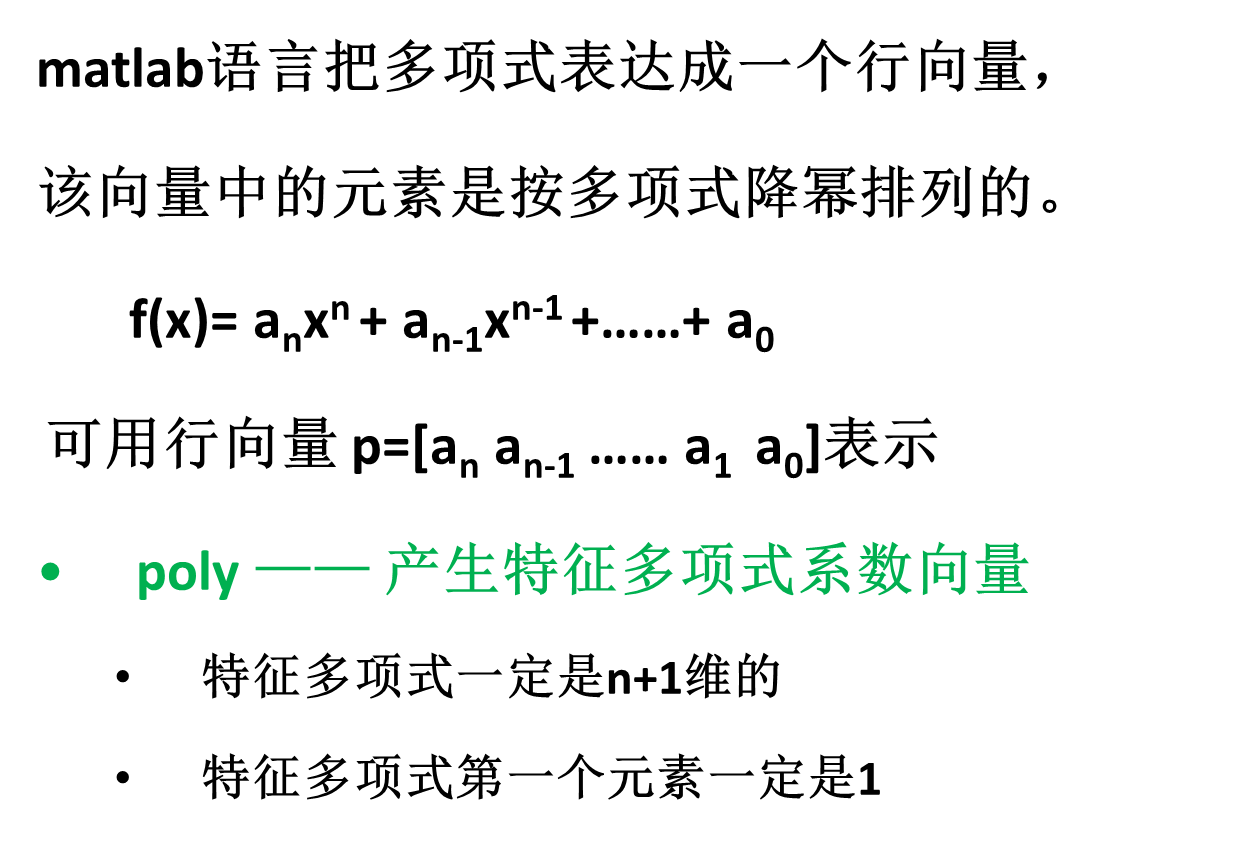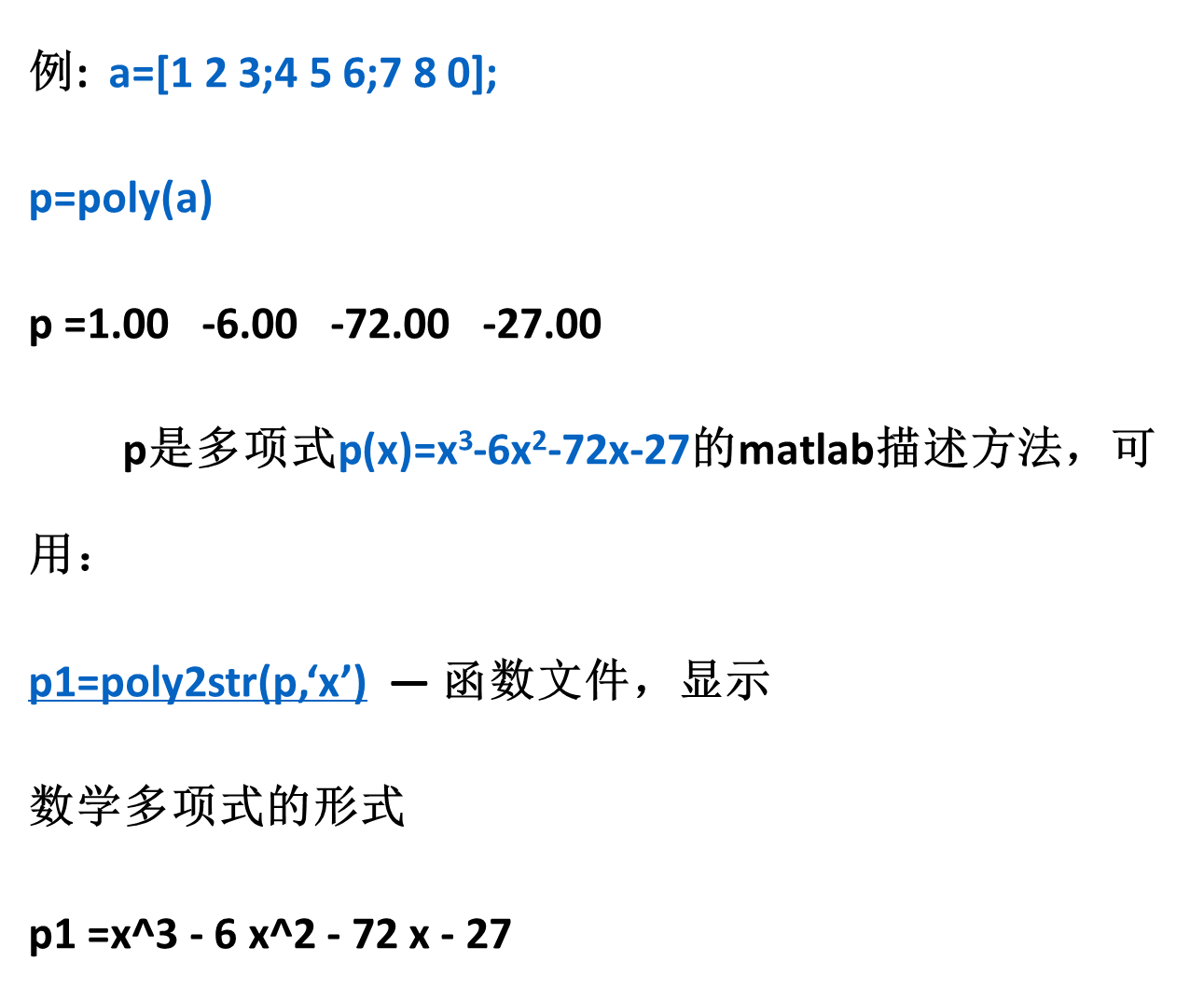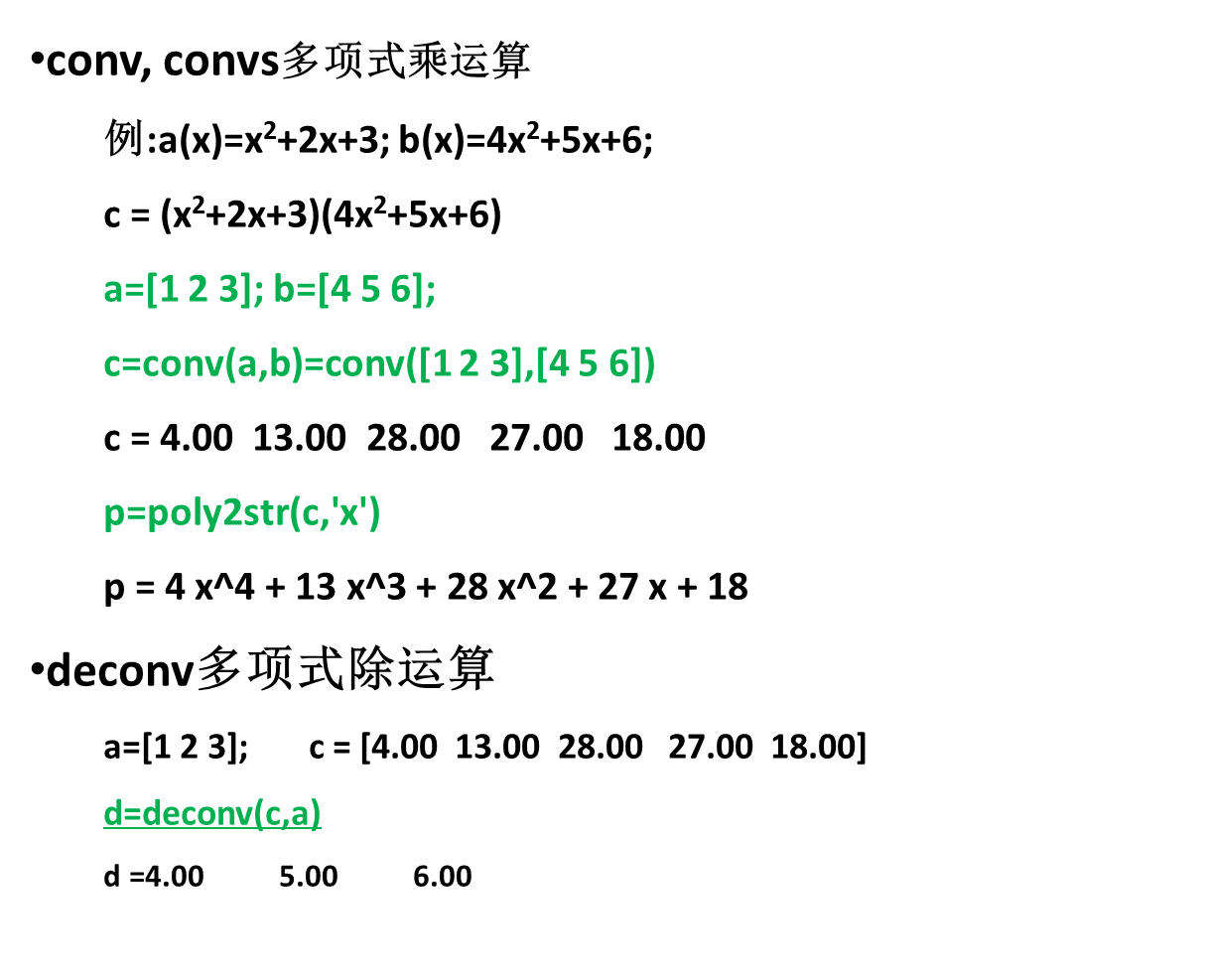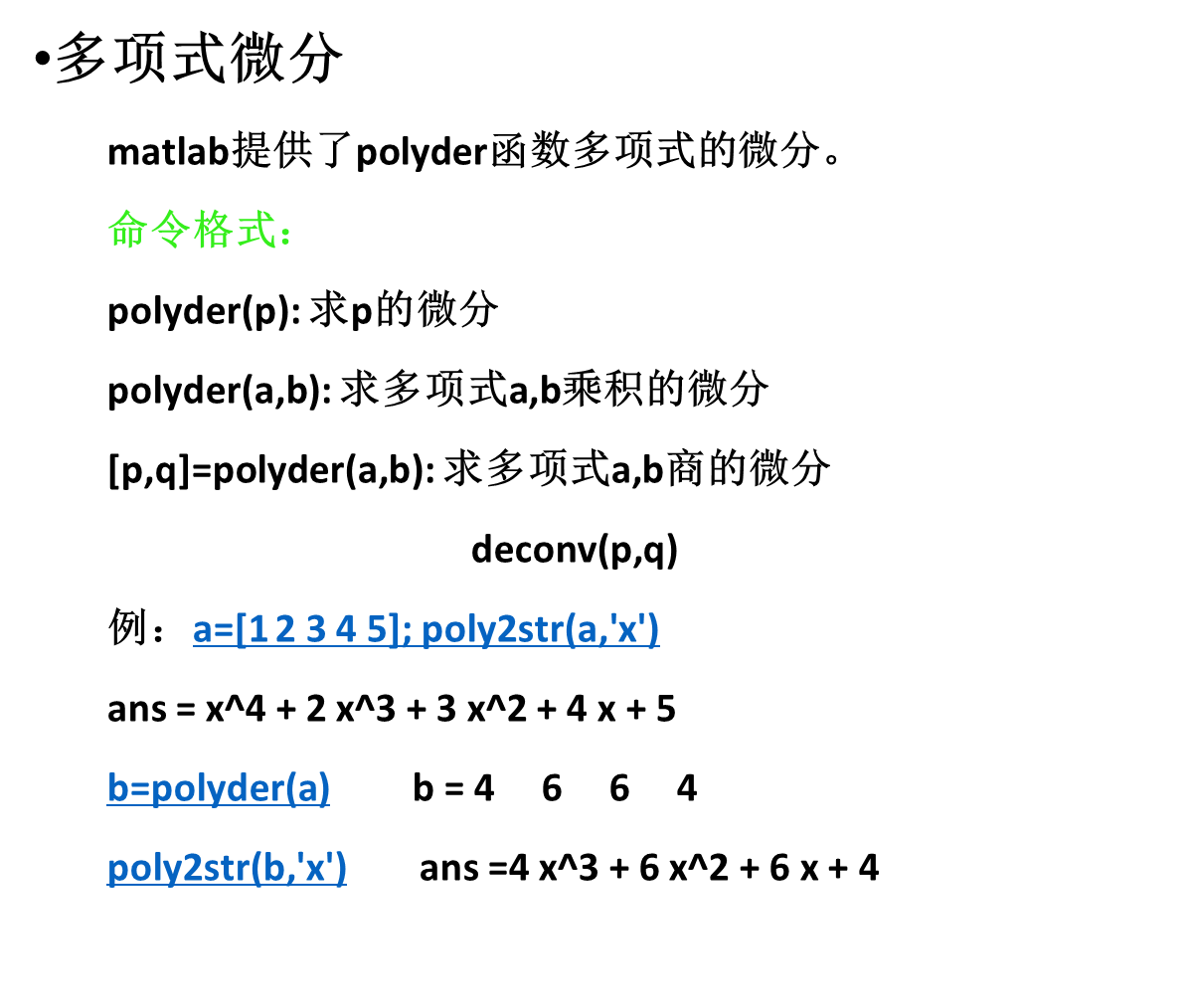# 1、MATLAB语言概述

## 1.1 MATLAB语言的发展

matlab语言是由美国的Clever Moler博士于1980年开发的
MATLAB = `Matrix Laboratory`

MATLAB是一种直译式的高级语言，比其它程序设计语言简单易用

## 1.3 MATLAB语言的功能

1. 强大的数值（矩阵）运算
2. 广泛的符号运算功能
3. 高级与低级兼备的图形功能
4. 计算结果的可视化
5. 可靠的容错功能
6. 应用灵活的兼容与接口功能
7. 信息量丰富的联机检索功能

### (1)矩阵运算功能

MATLAB提供了丰富的矩阵运算处理功能

C = A + B ，A,B,C都是矩阵,是矩阵的加运算

MATLAB主工具箱

## 1.4 MATLAB 语言基础

MATLAB中变量的命名规则是：
（1）变量名必须是不含空格的字符串；
（2）变量名以字母开头，之后可以是

（3）变量名区分大小写；
（4）变量名最多不超过19个字符；
MATLAB 的保留常量
eps, pi, NaN, Inf, i, j, i=sqrt(-1)
lastwarn, lasterr

### 例：单元(元胞)数组的创建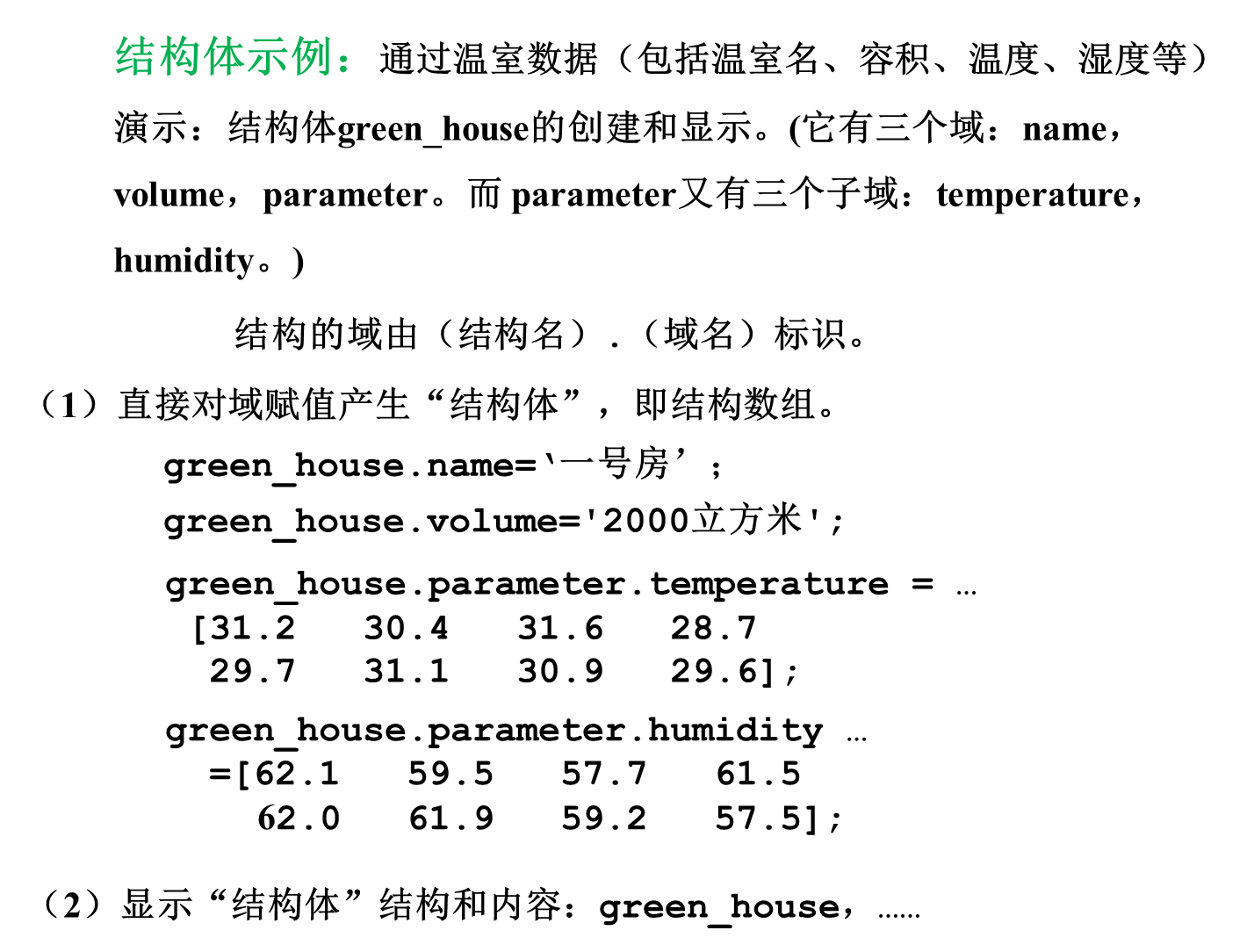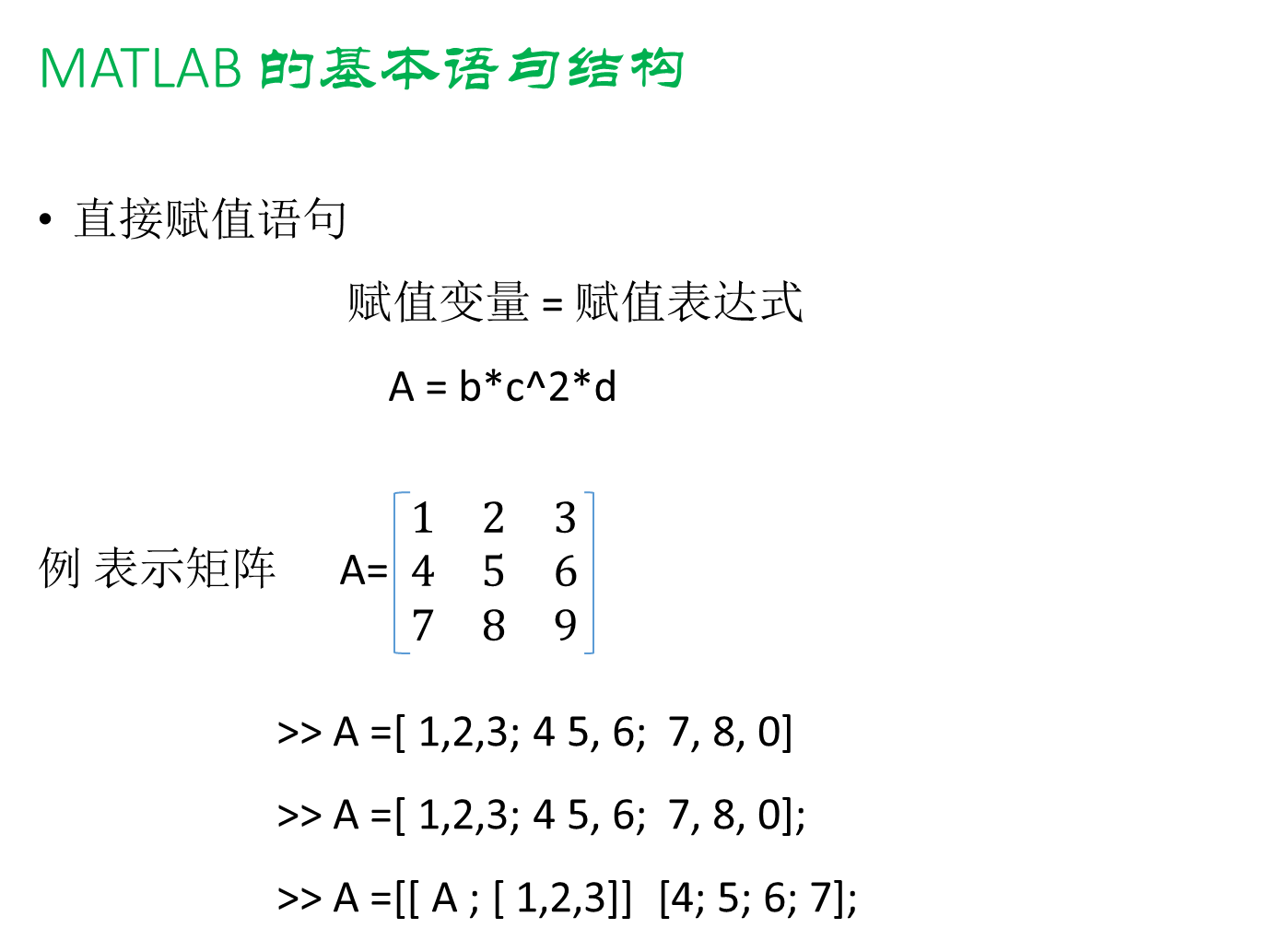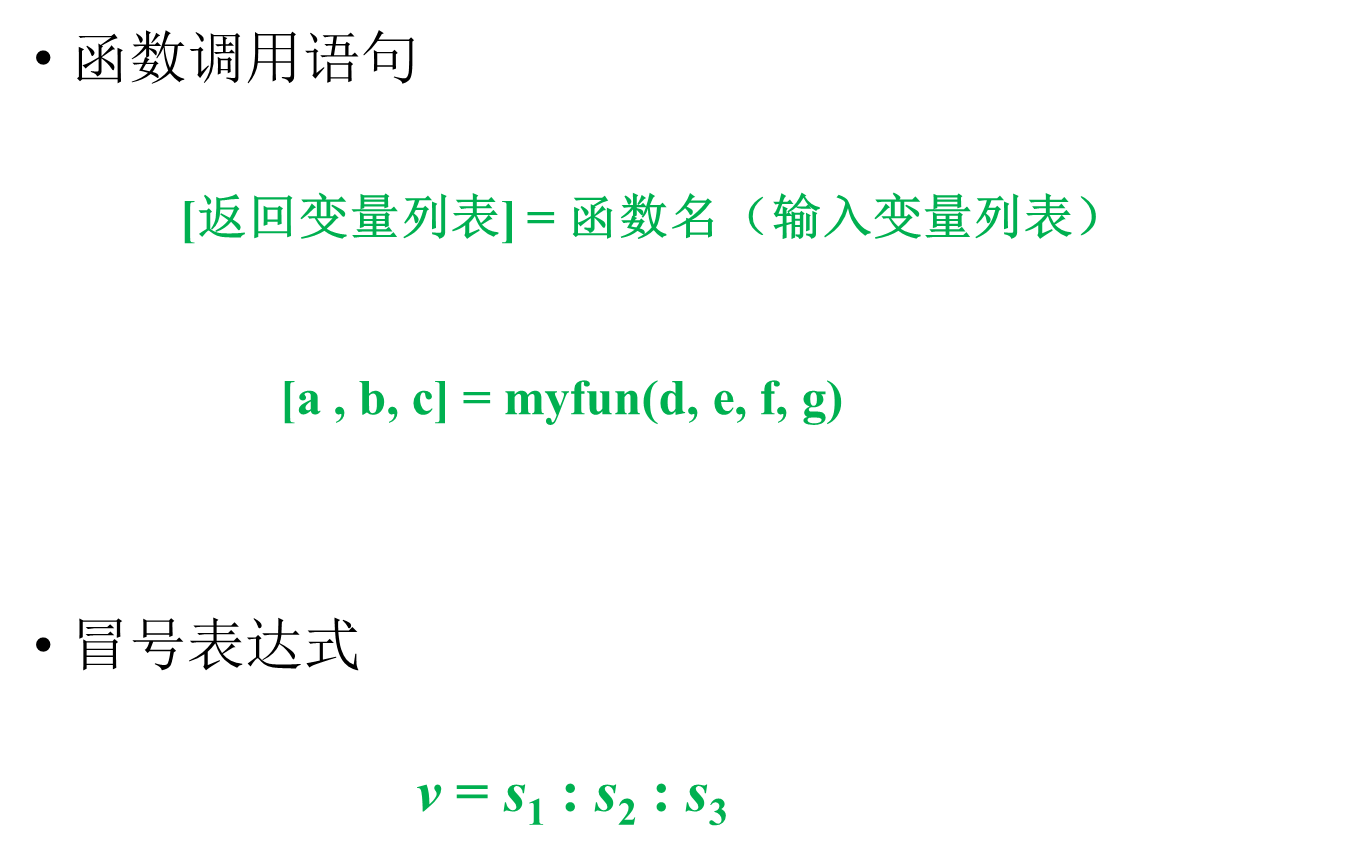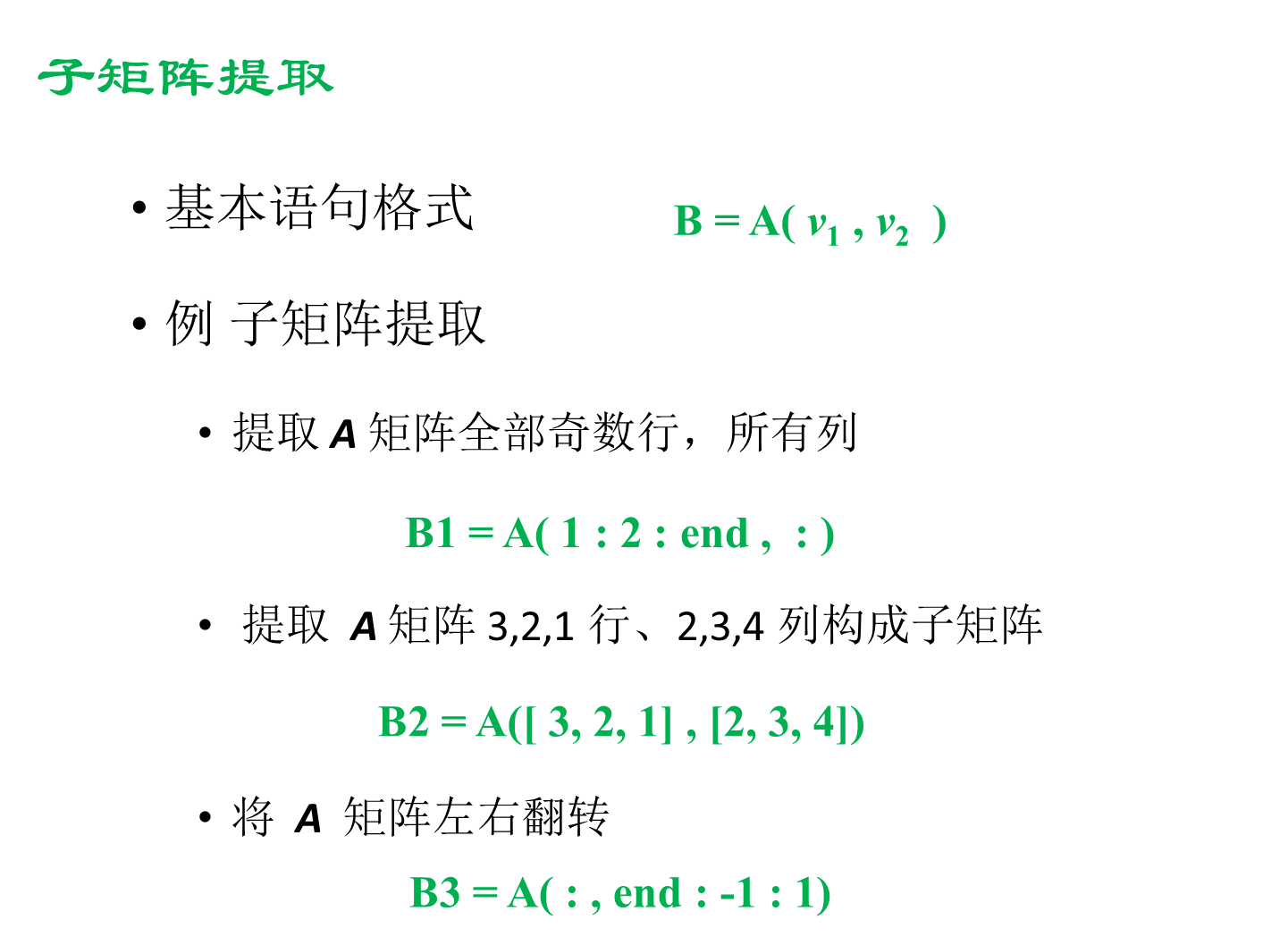# 2、MATLAB的基本运算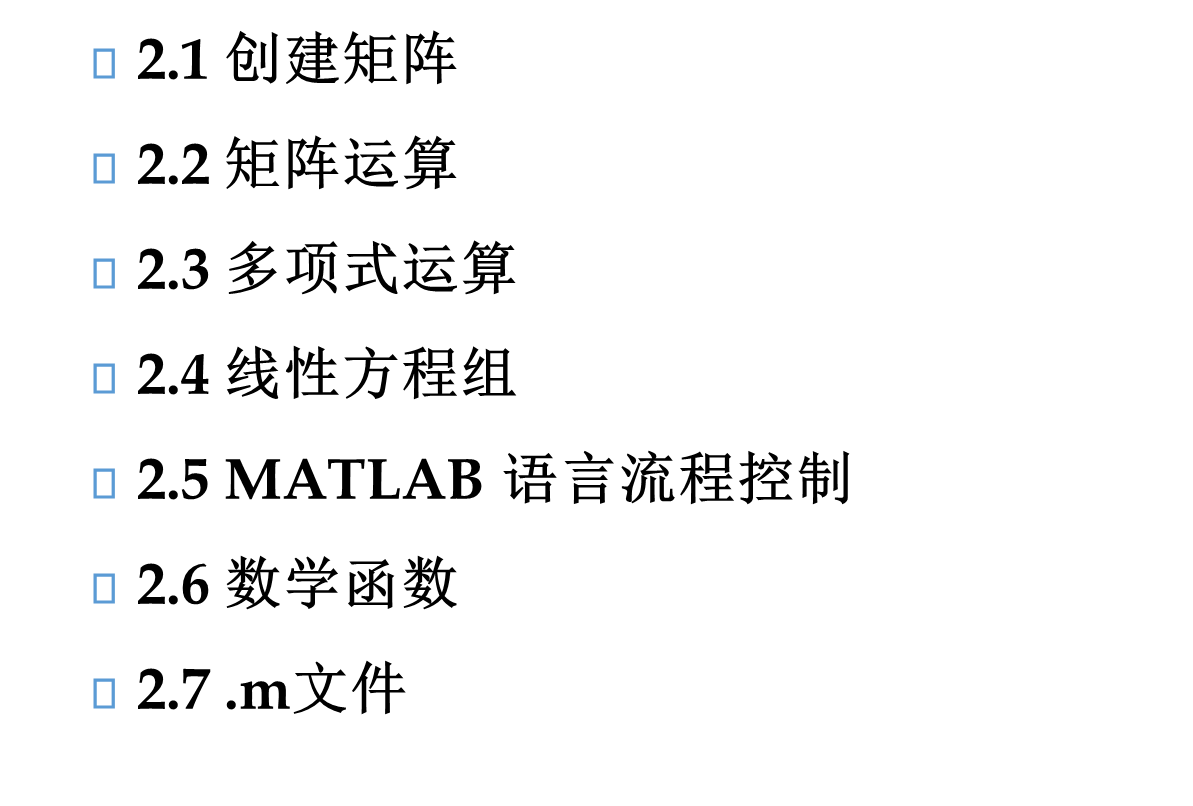## 2.1 创建矩阵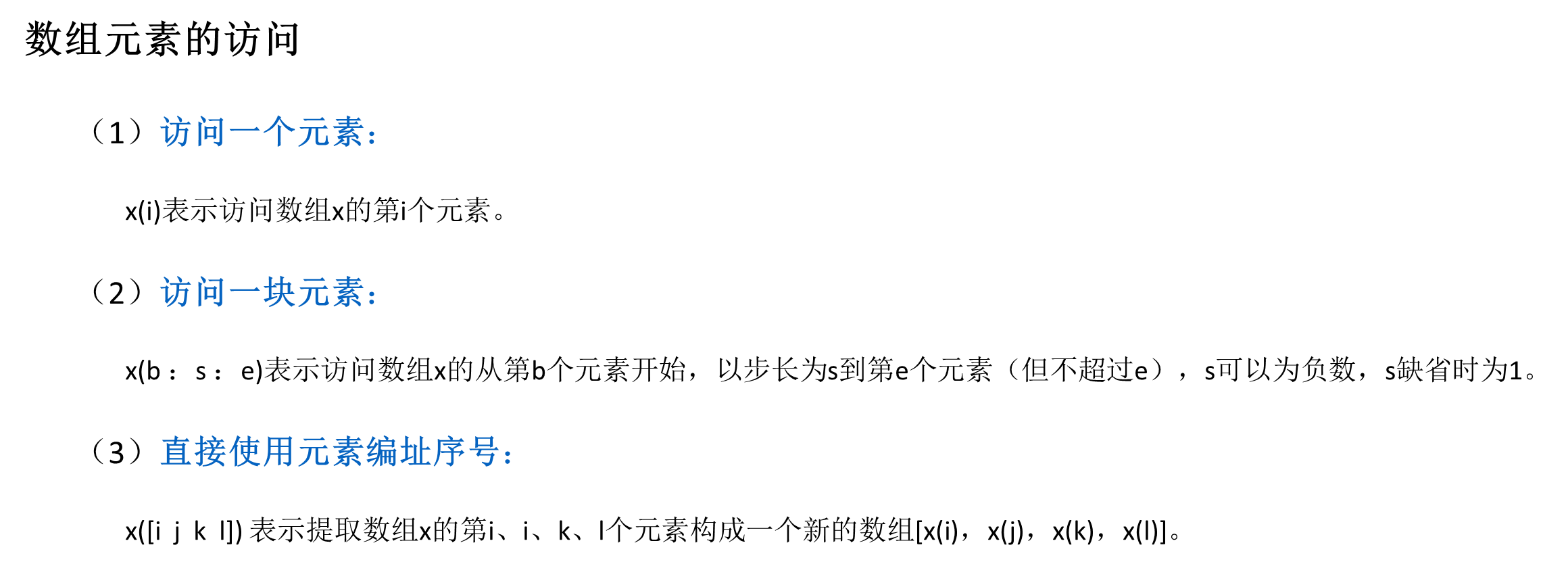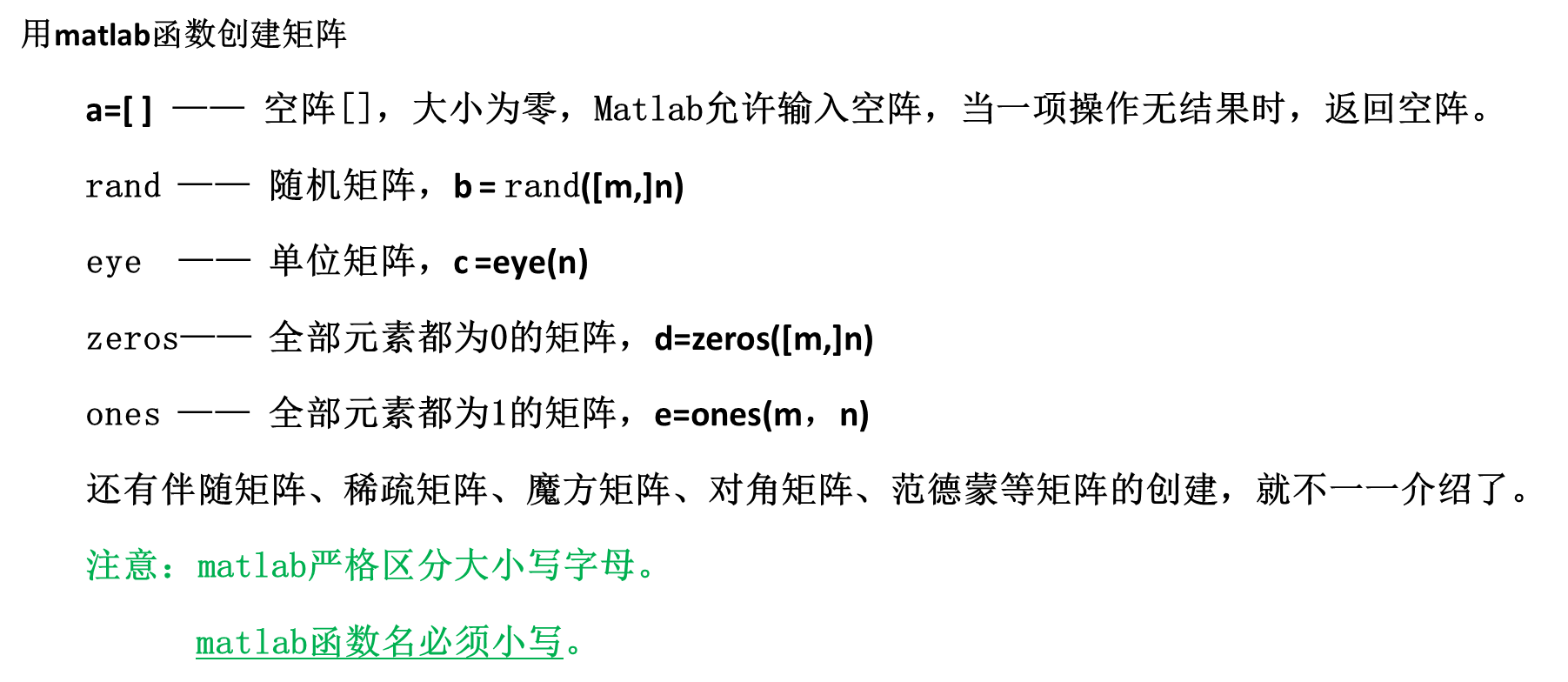## 2.2 矩阵运算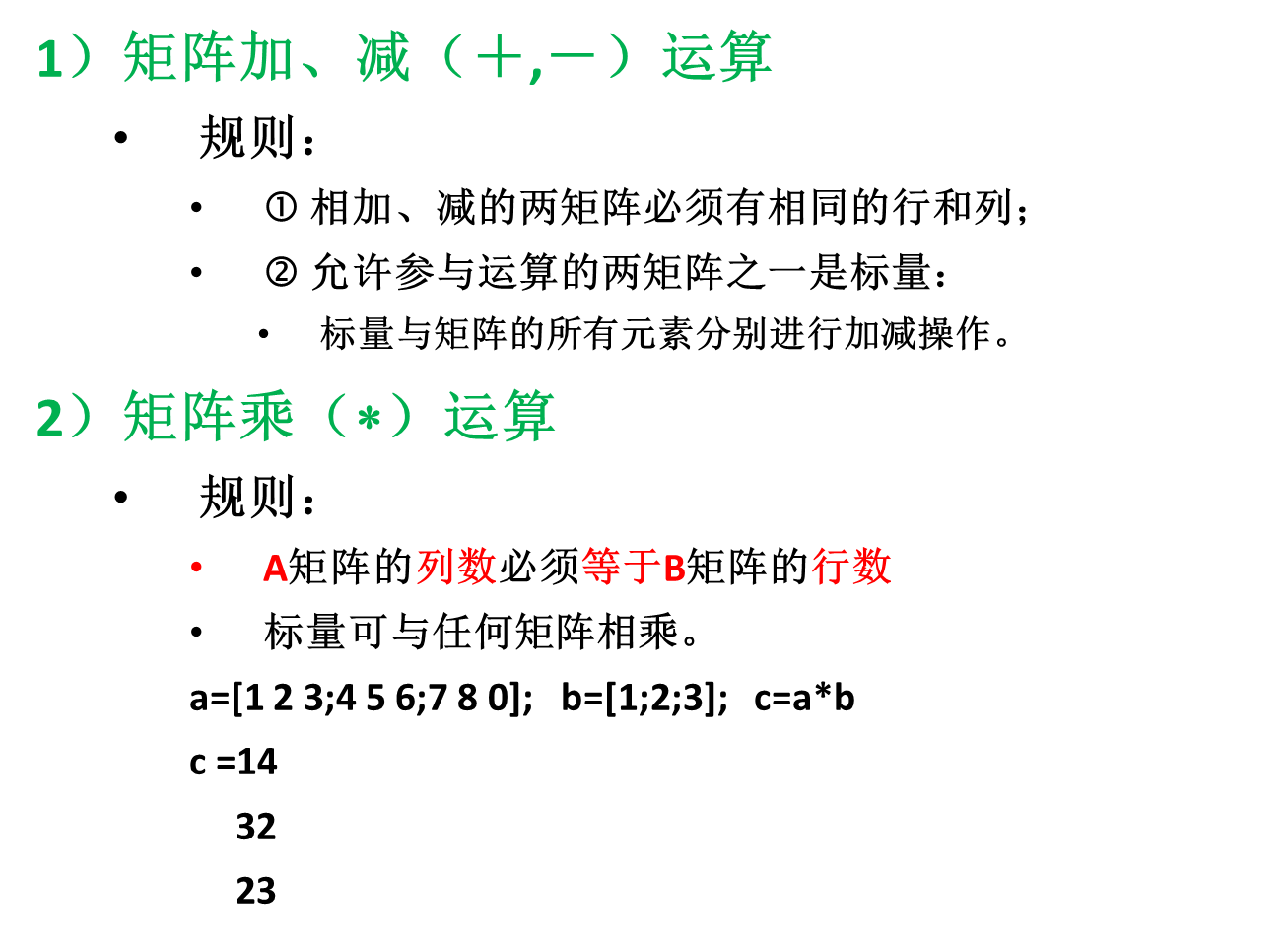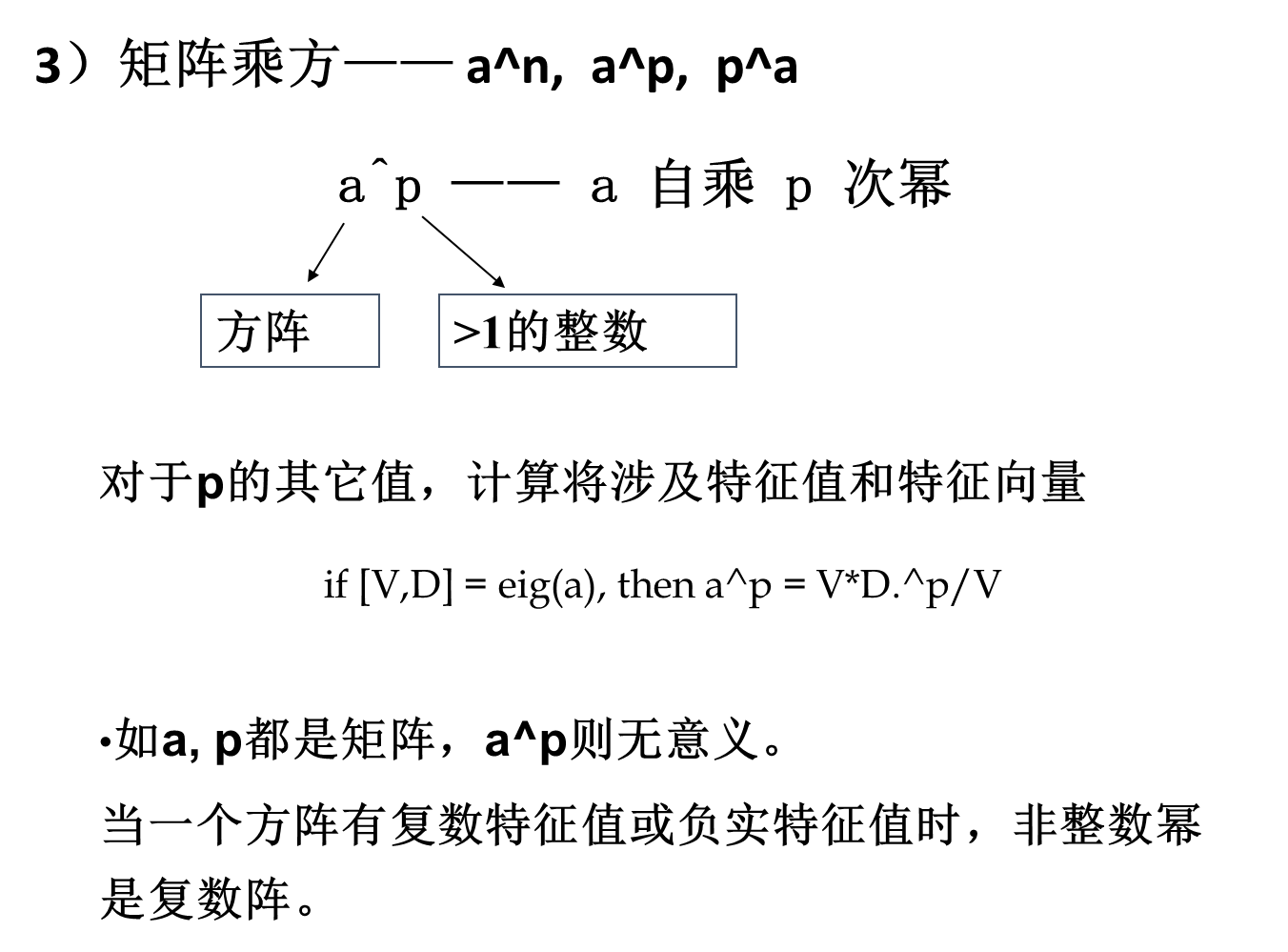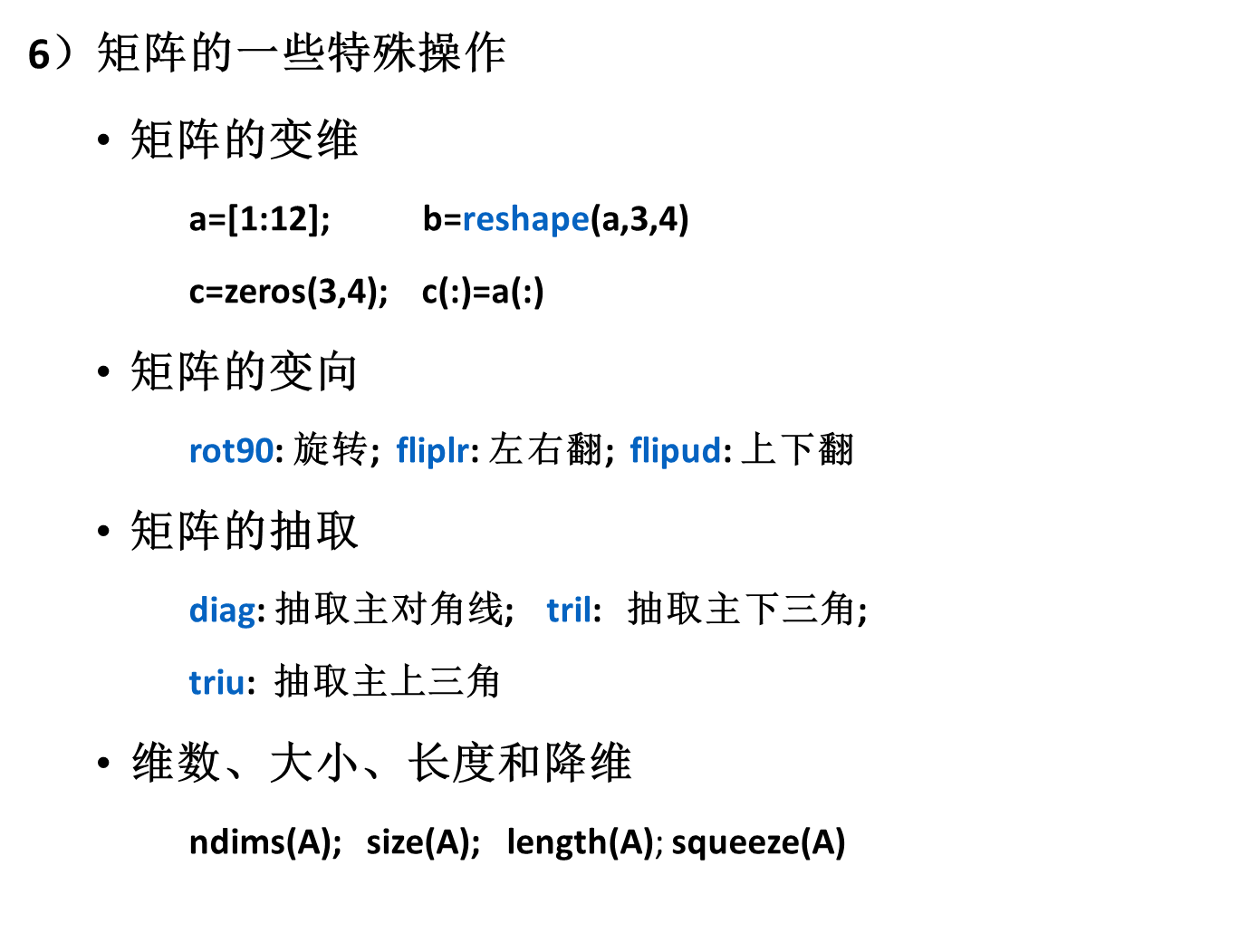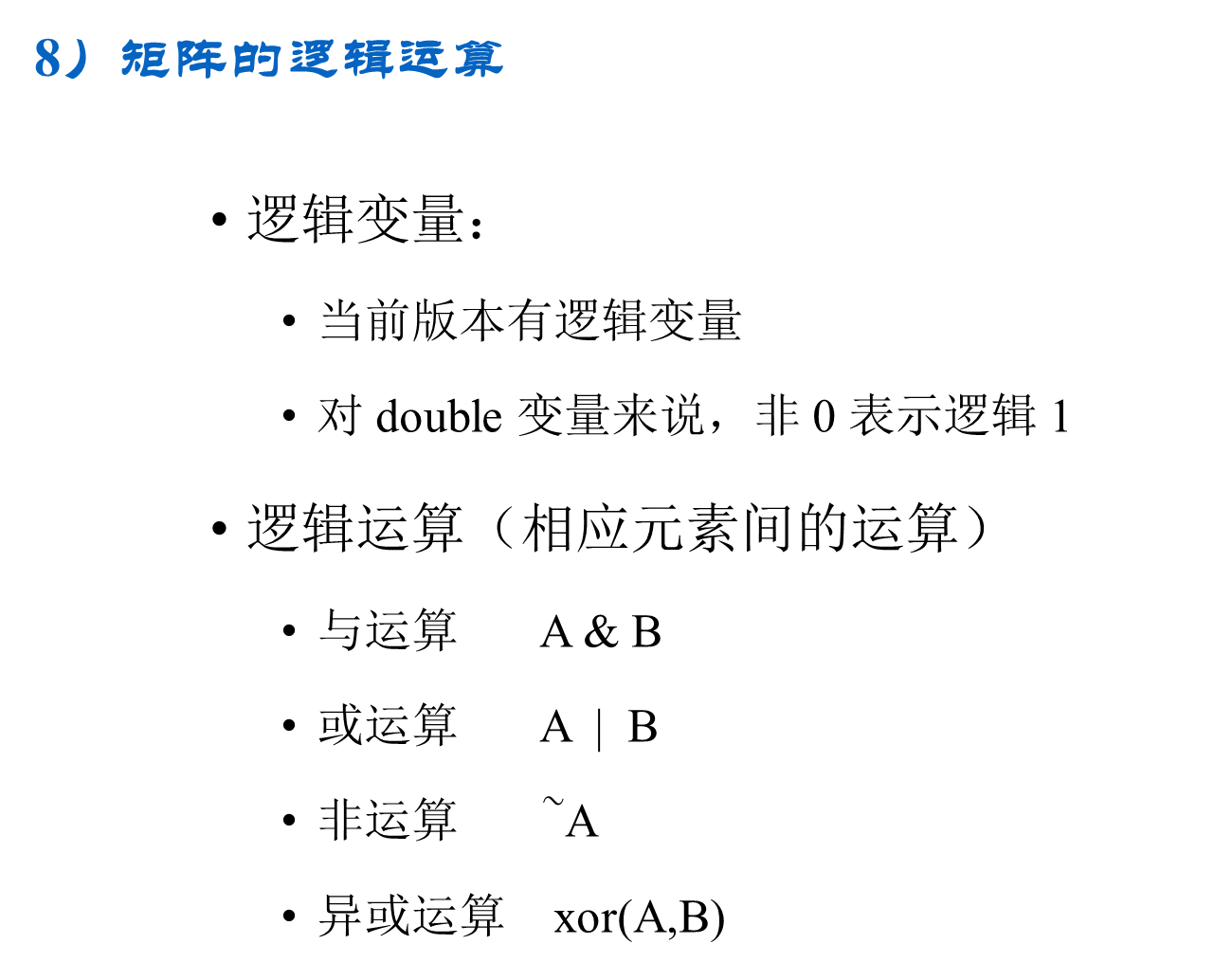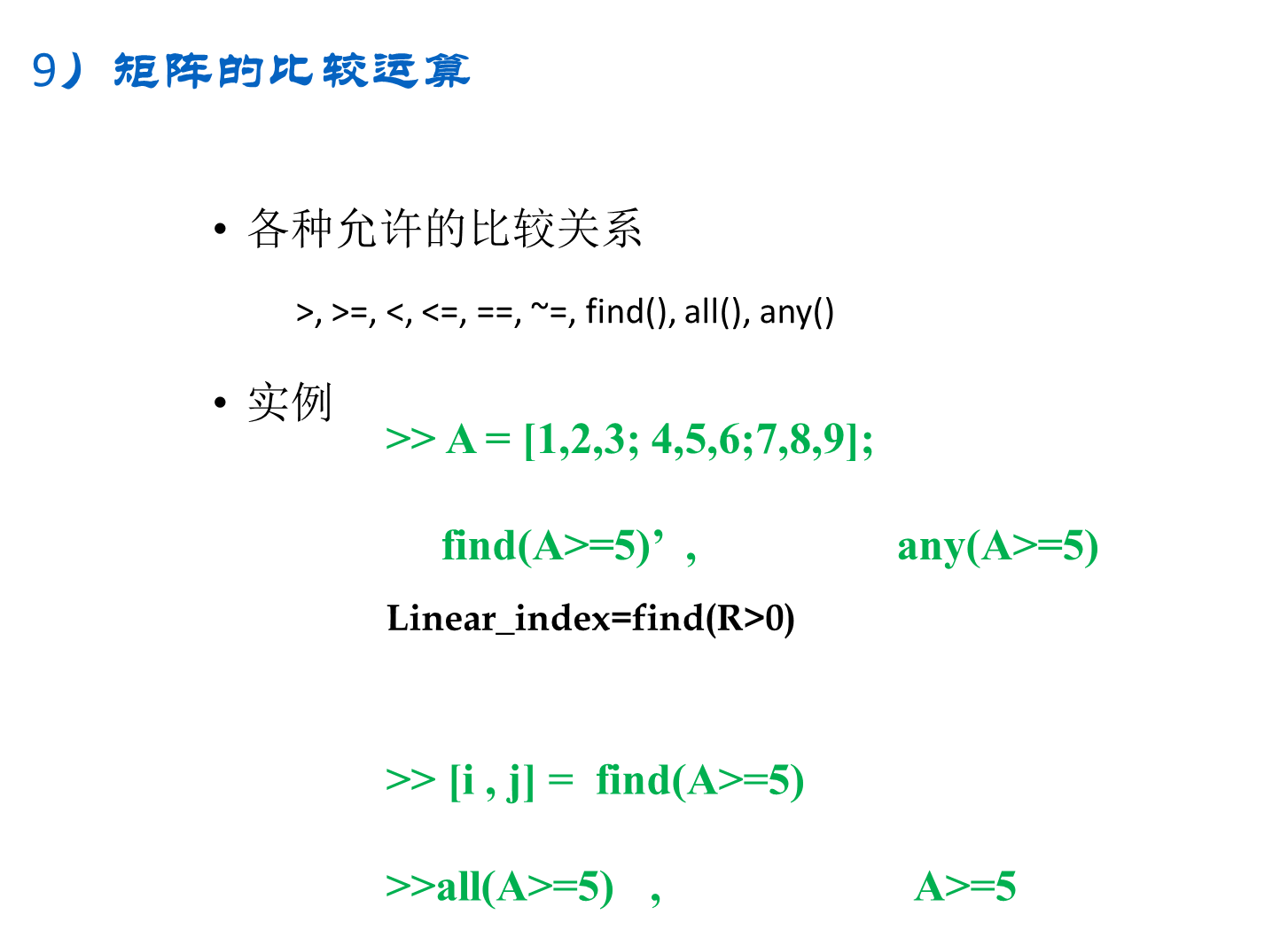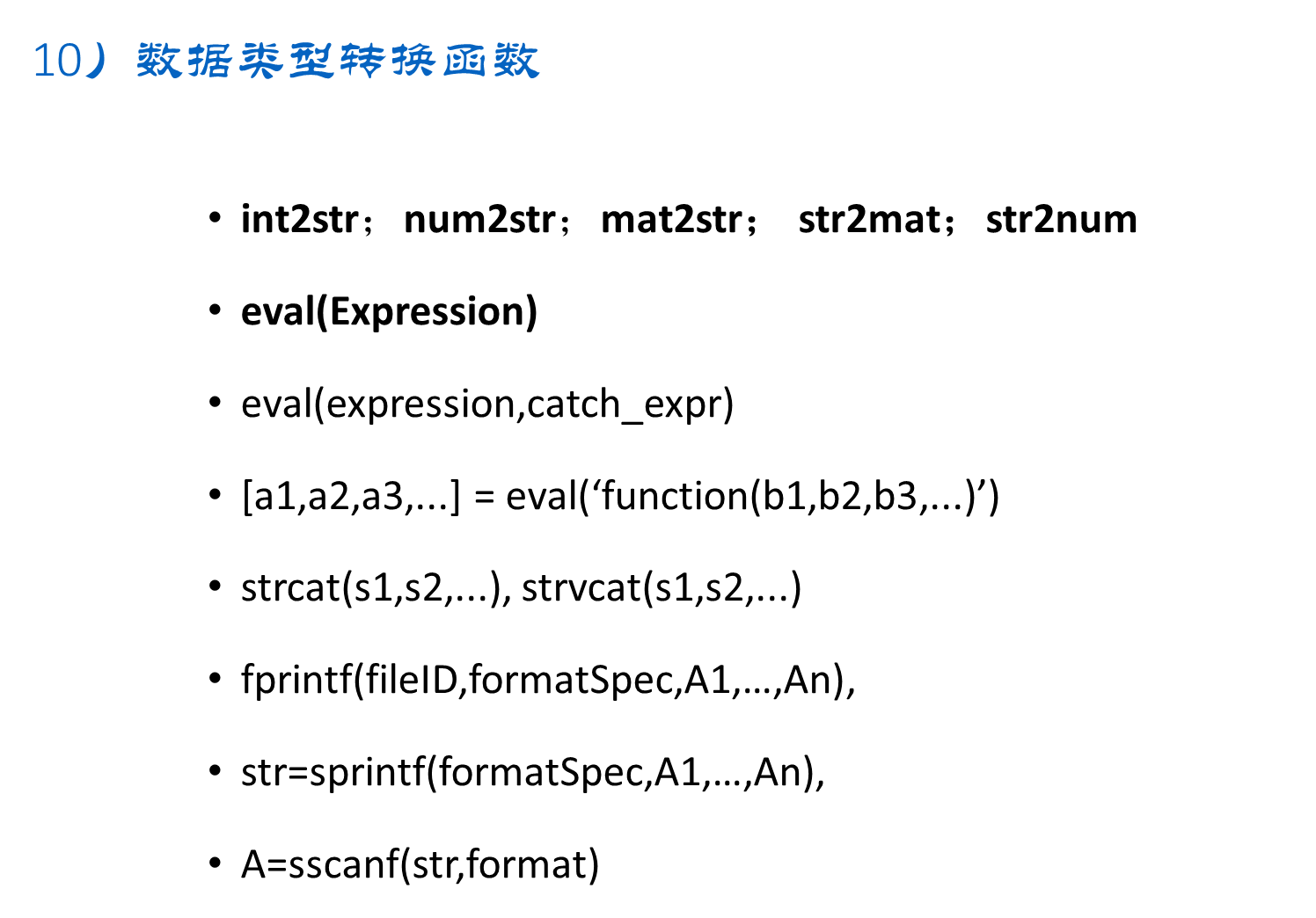## 2.3 多项式运算